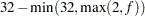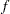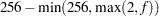CLASS Statement
CLASS variable <(options)><variable <(options)>> </ global-options> ;

The CLASS statement names the classification variables to be used as explanatory variables in the analysis. Response variables do not need to be specified in the CLASS statement.

The CLASS statement must precede the MODEL statement. Most options can be specified either as individual variable options or as global-options. You can specify options for each variable by enclosing the options in parentheses after the variable name. You can also specify global-options for the CLASS statement by placing them after a slash (/). Global-options are applied to all the variables specified in the CLASS statement. If you specify more than one CLASS statement, the global-options specified in any one CLASS statement apply to all CLASS statements. However, individual CLASS variable options override the global-options. You can specify the following values for either an option or a global-option:

CPREFIX=n

specifies that, at most, the first n characters of a CLASS variable name be used in creating names for the corresponding design variables. The default is, whereis the formatted length of the CLASS variable.

DESCENDING
DESC

reverses the sorting order of the classification variable. If both the DESCENDING and ORDER= options are specified, PROC GENMOD orders the categories according to the ORDER= option and then reverses that order.

LPREFIX=n

specifies that, at most, the first n characters of a CLASS variable label be used in creating labels for the corresponding design variables. The default is, whereis the formatted length of the CLASS variable.

MISSING

treats missing values (".", ".A", ..., ".Z" for numeric variables and blanks for character variables) as valid values for the CLASS variable.

ORDER=DATA | FORMATTED | FREQ | INTERNAL

specifies the sorting order for the levels of classification variables. This ordering determines which parameters in the model correspond to each level in the data, so the ORDER= option can be useful when you use the CONTRAST statement. By default, ORDER=FORMATTED. For ORDER=FORMATTED and ORDER=INTERNAL, the sort order is machine-dependent. When ORDER=FORMATTED is in effect for numeric variables for which you have supplied no explicit format, the levels are ordered by their internal values.

The following table shows how PROC GENMOD interprets values of the ORDER= option.

Value of ORDER=

Levels Sorted By

DATA

Order of appearance in the input data set

FORMATTED

External formatted values, except for numeric variables with no explicit format, which are sorted by their unformatted (internal) values

FREQ

Descending frequency count; levels with more observations come earlier in the order

INTERNAL

Unformatted value

For more information about sorting order, see the chapter on the SORT procedure in the Base SAS Procedures Guide and the discussion of BY-group processing in SAS Language Reference: Concepts.

PARAM=keyword

specifies the parameterization method for the classification variable or variables. You can specify any of the keywords shown in the following table; Design matrix columns are created from CLASS variables according to the corresponding coding schemes:

Value of PARAM=

Coding

EFFECT

Effect coding

GLM

Less-than-full-rank reference cell coding (this keyword can be used only in a global option)

ORDINAL
THERMOMETER

Cumulative parameterization for an ordinal CLASS variable

POLYNOMIAL
POLY

Polynomial coding

REFERENCE
REF

Reference cell coding

ORTHEFFECT

Orthogonalizes PARAM=EFFECT coding

ORTHORDINAL
ORTHOTHERM

Orthogonalizes PARAM=ORDINAL coding

ORTHPOLY

Orthogonalizes PARAM=POLYNOMIAL coding

ORTHREF

Orthogonalizes PARAM=REFERENCE coding

All parameterizations are full rank, except for the GLM parameterization. The REF= option in the CLASS statement determines the reference level for EFFECT and REFERENCE coding and for their orthogonal parameterizations.

If PARAM=ORTHPOLY or PARAM=POLY and the classification variable is numeric, then the ORDER= option in the CLASS statement is ignored, and the internal unformatted values are used. See the section Other Parameterizations of Chapter 19, Shared Concepts and Topics, for further details.

REF=’level’ | keyword

specifies the reference level for PARAM=EFFECT, PARAM=REFERENCE, and their orthogonalizations. For an individual (but not a global) variable REF= option, you can specify the level of the variable to use as the reference level. Specify the formatted value of the variable if a format is assigned. For a global or individual variable REF= option, you can use one of the following keywords. The default is REF=LAST.

FIRST

designates the first ordered level as reference.

LAST

designates the last ordered level as reference.

TRUNCATE<=n>

specifies the length n of CLASS variable values to use in determining CLASS variable levels. The default is to use the full formatted length of the CLASS variable. If you specify TRUNCATE without the length, the first 16 characters of the formatted values are used. When formatted values are longer than 16 characters, you can use this option to revert to the levels as determined in releases before SAS 9. The TRUNCATE option is available only as a global option.

### Class Variable Naming Convention

Parameter names for a CLASS predictor variable are constructed by concatenating the CLASS variable name with the CLASS levels. However, for the POLYNOMIAL and orthogonal parameterizations, parameter names are formed by concatenating the CLASS variable name and keywords that reflect the parameterization. See the section Other Parameterizations in Chapter 19, Shared Concepts and Topics, for examples and further details.

### Class Variable Parameterization with Unbalanced Designs

PROC GENMOD initially parameterizes the CLASS variables by looking at the levels of the variables across the complete data set. If you have an unbalanced replication of levels across variables or BY groups, then the design matrix and the parameter interpretation might be different from what you expect. For instance, suppose you have a model with one CLASS variable A with three levels (1, 2, and 3), and another CLASS variable B with two levels (1 and 2). If the third level of A occurs only with the first level of B, if you use the EFFECT parameterization, and if your model contains the effect A(B) and an intercept, then the design for A within the second level of B is not a differential effect. In particular, the design looks like the following:

Design Matrix

A(B=1)

A(B=2)

B

A

A1

A2

A1

A2PROC GENMOD detects linear dependency among the last two design variables and sets the parameter for A2(B2) to zero, resulting in an interpretation of these parameters as if they were reference- or dummy-coded. The REFERENCE or GLM parameterization might be more appropriate for such problems.